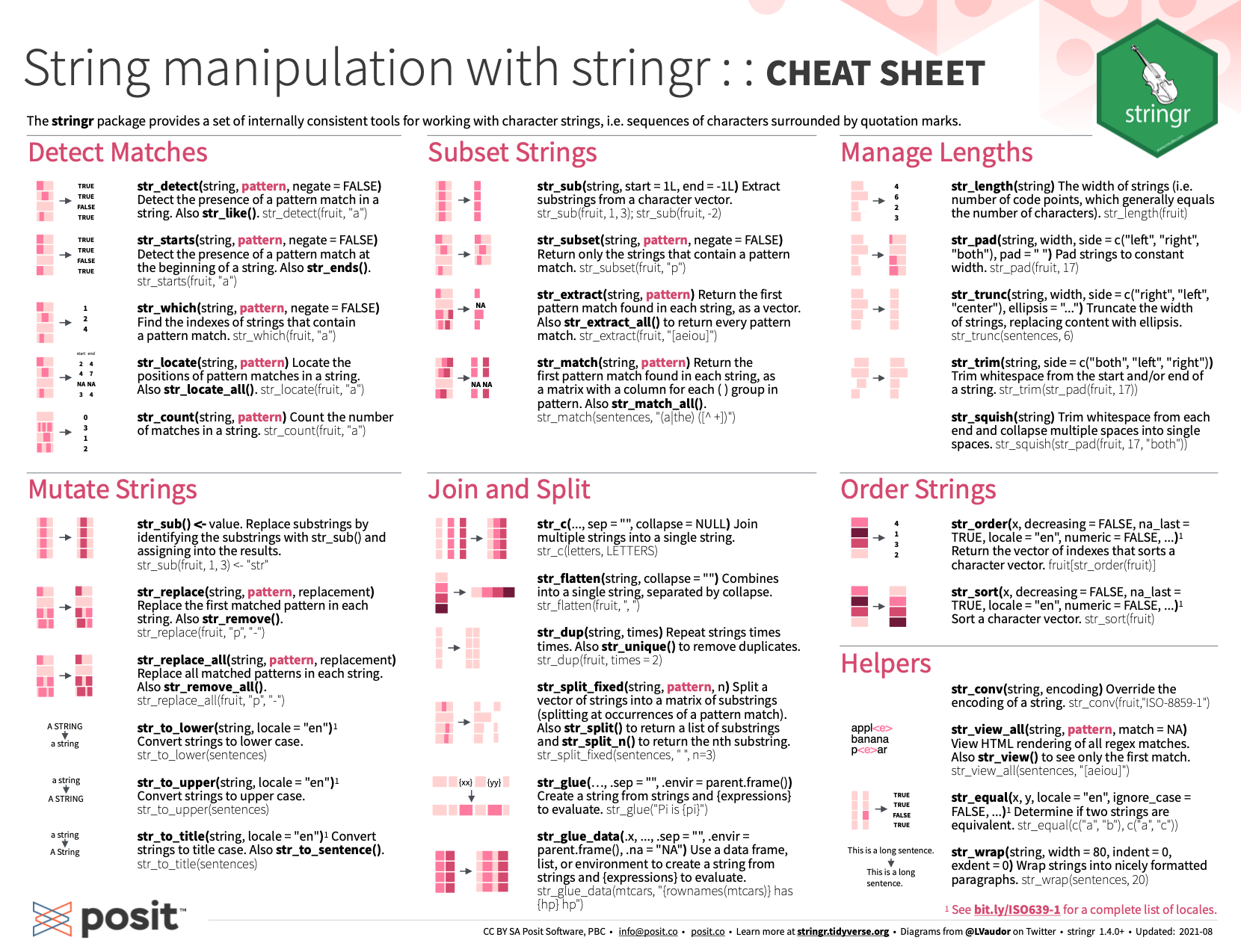## 9.6 SummaryThis chapter began by explaining how to define text — or data of type character — in R (Section 9.2). The bulk of the chapter then covered various tools for tackling basic and more advanced tasks of text-manipulation (Sections 9.3 and 9.4), before providing a glimpse of possible applications (Section 9.5).

As a summary, Table 9.4 repeats the overview from Section 9.2.4. At this point, we are familiar with the base R commands for tackling so-called basic tasks (see Sections 9.3) and the stringr commands for addressing the more advanced tasks (see Sections 9.4).

Table 9.4: Basic and advanced tasks of text-manipulation (involving a string s and pattern p).
Task R base stringr
Measure the length of strings s: nchar(s) str_length(s)
Change chars in s to lower case: tolower(s) str_to_lower(s)$$^{2}$$
Change chars in s to upper case: toupper(s) str_to_upper(s)$$^{2}$$
Combine or collapse strings ...: paste(...)$$^{1}$$ str_c(...)
Split a string s: strsplit(s, split) str_split(s, split)$$^{2}$$
Sort a character vector s: sort(s) str_sort(s)$$^{2}$$
Extract or replace substrings in s: substr(s, start, stop)$$^{1}$$ str_sub(s, start, stop)
Translate old into new chars in s: chartr(old, new, s)
– Text as input or output: print(), cat(), format(), readLines(), scan(), writeLines()$$^{1}$$
View strings in s that match p: str_view(s, p)$$^{a}$$
Detect pattern p in strings s: grep(p, s) grepl(p, s)$$^{1}$$ str_detect(s, p)
Locate pattern p in strings s: gregexpr(p, s)$$^{1}$$ str_locate(s, p)$$^{a}$$
Obtain strings in s that match p: grep(p, s, value = TRUE)$$^{1}$$ str_subset(s, p)
Extract matches of p in strings s: regmatches(s, gregexpr(p, s))$$^{1}$$ str_extract(s, p)$$^{a}$$
Replace matches of p by r in s: gsub(p, r, s)$$^{1}$$ str_replace(s, p, r)$$^{a}$$
Count matches of p in strings s: str_count(s, p)

#### Table notes

• $$^{1}$$: base R functions with additional variants that tweak their functionality (see their documentation).

• $$^{2}$$: stringr functions with additional variants that tweak their functionality.

• $$^{a}$$: stringr functions with an additional suffix _all() that applies the function to all matches (rather than just to the first match).

The frequent reference to a pattern p in the table shows that much of the power of functions addressing the more advanced tasks is based on R’s ability to use regular expressions for pattern matching. (See Appendix E for a primer on using regular expressions.)

After working through this chapter, you are able to:

1. understand that text consists of characters and comes in strings,
2. enter character data and rare symbols as Unicode characters,
3. distinguish between different tasks to solve with text,
4. use base R commands to define, combine, and manipulate strings of text,
5. read and write basic regular expressions (see Appendix E),
6. use stringr commands to detect, locate, extract, replace, and count patterns in strings of text.

For an overview of text manipulation with stringr and using regular expressions, take a look at the RStudio cheatsheet to check which commands you are now familiar with and which others you can still discover in the future:Figure 9.7: Text and string manipulation with stringr and regular expressions
from RStudio cheatsheets.

The contributed cheatsheets also contain a fine reference on Basic Regular Expressions in R.

Let’s test our knowledge and skills on manipulating strings of text by completing the following exercises.